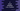# 4 different C programs to check if a number is prime or not## C program to check if a number is prime or not:

In this post, we will learn how to write a C program to check if a given number is prime or not. The program will take one number as an input from the user and print a message that it is prime or not prime.

### What is a Prime number:

A number is called a prime number if the number is greater than 1 and it is divisible by 1 and the number itself. For example, 5, 7, 11 etc. are prime numbers.

We will use a loop to check if the number is divisible by any other number. The loop will start from 2. If it is not divisible by any other number, it is a prime number.

### Method 1: C program to check if a number is prime or not:

In this method, we will run one loop to iterate from i to number and on iteration, it will check if the current value of the loop can divide the number or not. If it can divide, it is not a prime number. Else, it will be a prime number.

``````#include <stdio.h>

int main()
{
int number, isPrime = 1;

printf("Enter a number: ");
scanf("%d", &number);

if (number == 1)
{
isPrime = 0;
}
for (int i = 2; i < number; i++)
{
if (number % i == 0)
{
isPrime = 0;
break;
}
}

if (isPrime)
{
printf("%d is a prime number", number);
}
else
{
printf("%d is not a prime number", number);
}
}``````

Here,

• The integer variable number is used to keep the user-input number. isPrime is another integer variable to hold if the number is prime or not. It will be 1 if the number is prime, else it will be 0.
• Before the loop starts, it checks if the number is 1. If yes, it assigns 0 to isPrime.
• The for loop runs from i = 2 to i = number - 1. On each iteration, it checks if the number is divisible by the current value of i or not. If it is true for any value of i, it assigns 0 to isPrime and exits from the loop as the number is not a prime number.
• Once the loop ends, it checks the value of isPrime and prints one message.

If you run this program, it will print outputs as below:

``````Enter a number: 1
1 is not a prime number

Enter a number: 2
2 is a prime number

Enter a number: 97
97 is a prime number

Enter a number: 98
98 is not a prime number``````

### Method 2: C program to check if a number is prime or not by iterating to number/2:

We can also iterate to number/2 instead of number. It will reduce the iteration by half. So, let’s change the above program to iterate from 2 to number/2.

``````#include <stdio.h>

int main()
{
int number, isPrime = 1;

printf("Enter a number: ");
scanf("%d", &number);

if (number == 1)
{
isPrime = 0;
}
for (int i = 2; i <= number/2; i++)
{
if (number % i == 0)
{
isPrime = 0;
break;
}
}

if (isPrime)
{
printf("%d is a prime number", number);
}
else
{
printf("%d is not a prime number", number);
}
}``````

It will print similar results.

### Method 3: C program to check if a number is prime or not by iterating to square root of the number:

We can reduce the number of iterations of the loop even further by iterating to the square root of the number. The math.h header file provides a method called sqrt to find the square root of a number. Let’s use it to write the above program:

``````#include <stdio.h>
#include <math.h>

int main()
{
int number, isPrime = 1;

printf("Enter a number: ");
scanf("%d", &number);

if (number == 1)
{
isPrime = 0;
}
for (int i = 2; i <= sqrt(number); i++)
{
if (number % i == 0)
{
isPrime = 0;
break;
}
}

if (isPrime)
{
printf("%d is a prime number", number);
}
else
{
printf("%d is not a prime number", number);
}
}``````

You will get a similar result.

### Method 4: C program to check if a number is prime or not by using a separate method:

Let’s use a separate method to check if a number is prime or not. This method will take one number as its parameter and return 1 if it is a prime number or 0 if it is not.

``````#include <stdio.h>
#include <math.h>

int isPrime(int number)
{
if (number == 1)
{
return 0;
}
for (int i = 2; i <= sqrt(number); i++)
{
if (number % i == 0)
{
return 0;
}
}
return 1;
}

int main()
{
int number;

printf("Enter a number: ");
scanf("%d", &number);

if (isPrime(number))
{
printf("%d is a prime number", number);
}
else
{
printf("%d is not a prime number", number);
}
}``````

Here,

• isPrime method is used to check if a number is prime or not. It returns 1 if the number is prime. Else, it returns 0.
• If the number is 1, it returns 0, i.e. it is not a prime number.
• The for loop runs from 2 to square root of the number. If the number is divisible by any value in the loop, it returns 0.
• It return 1 if the for loop ends, i.e. the number is not divisible by any number.

It will give similar output.